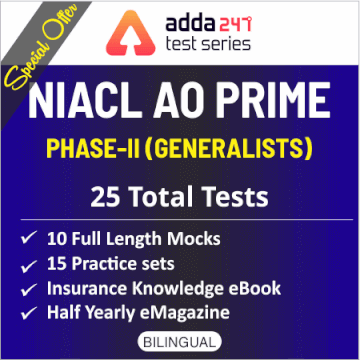Dear Aspirants,

### Quantitative Aptitude Quiz For IBPS PO/Clerk Prelims

Numerical Ability or Quantitative Aptitude Section has given heebie-jeebies to the aspirants when they appear for a banking examination. As the level of every other section is only getting complex and convoluted, there is no doubt that this section, too, makes your blood run cold. The questions asked in this section are calculative and very time-consuming. But once dealt with proper strategy, speed, and accuracy, this section can get you the maximum marks in the examination. Following is the Quantitative Aptitude quiz to help you practice with the best of latest pattern questions.
WATCH VIDEO SOLUTION HERE

Directions (1-5): Given below is the bar graph which shows the number of Desktop and Laptops sold by four different shops A, B, C and D.

Q1. What is the average number of laptops sold by store B, C and D.
1750
1700
1850
1900
1800
Q2. Desktops sold by store A is what percent more than Laptops sold by store C (approximately)?
66%
120%
175%
133%
166%
Q3. Total Laptops sold by store B and C together are how much more or less than total Desktops sold by store C and D together?
2100
2200
2150
1500
1800
Solution:
Required number = (2400 + 3000) – (1800 + 1500)
= 2100

Q4. What is the ratio of desktops sold by store A and C together to total Laptops sold by stores B and D together?
32 : 23
33 : 19
32 : 21
33 : 20
32 : 19
Q5. Laptops sold by store B is what percent more or less than Laptops sold by store C.
Q6. A is 50% more efficient than B. They together start the work and 7/12th of work is left after 8 days. Find in what time B alone will complete the whole work.
36 days
44 days
50 days
40 days
48 days
Q7. The circumference of two circles is 132 m and 176 m respectively. What is difference between the area of larger circle and smaller circle ? (in m²)
1052
1128
1258
1078
1528
Q8. Average of four numbers is 26. Sum of the smallest and the largest number is 55. If difference between other two numbers is one then find the square of second smallest number.
576
625
529
484
676
Solution:
Let four numbers in increasing order is a, b, c & d
ATQ,
a + b + c + d = 4 x 26= 104
a +d = 55
⇒ b + c = 49
c – b = 1
b = 24, c= 25
b² = 576

Q9. Mixture containing milk and water in ratio of 2 : 3 has volume of 30 liters. If 10 L of water is added to it then find the ratio of milk to water in the mixture?
7 : 9
3 : 7
3 : 5
2 : 3
5 : 7
Q10. Shopkeeper buys 3 articles for Rs 2100 (CP of each article is same). If he made overall profit of 20% on selling all the three articles then find the profit obtained on selling one article.
Rs 140
Rs 170
Rs 280
Rs 70
Rs 105
Directions (11-15): In each of these questions, two equations numbered I and II are given. You have to solve both the equation and give answer

Q11. I. 2x² + 11x + 15 = 0
II. 4y² + 13y + 9 = 0
if x < y
if x ≤ y
if x > y
if x ≥ y
if x = y or the relationship cannot be established
Q12. I. x² - 36x + 324 = 0
II. y² - 35y + 216 = 0
if x < y
if x ≤ y
if x > y
if x ≥ y
if x = y or the relationship cannot be established
Q13.

if x < y
if x ≤ y
if x > y
if x ≥ y
if x = y or the relationship cannot be established
Q14. I. 2x² + 17x + 35 = 0
II. 3y² + 17y + 24 = 0
if x < y
if x ≤ y
if x > y
if x ≥ y
if x = y or the relationship cannot be established
Q15. I. x² + 72 = 108
II. y³ + 581 = 365
if x < y
if x ≤ y
if x > y
if x ≥ y
if x = y or the relationship cannot be established
Solution:
I. x² + 72 = 108
x² = 108 – 72 = 36
x = ±6
II. y³ + 581 = 365
y³ = –216
y = –6
x ≥ y

You May also like to Read: